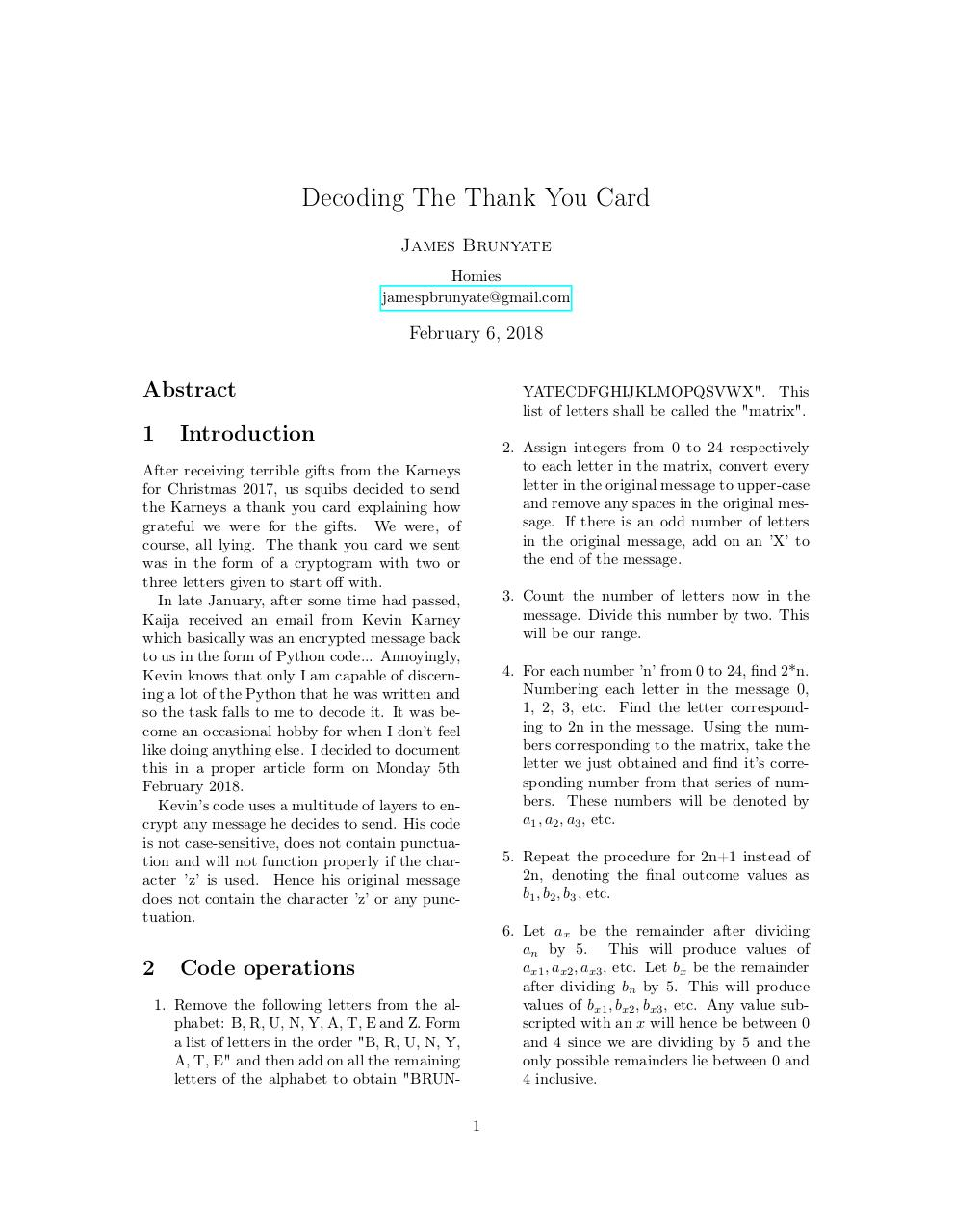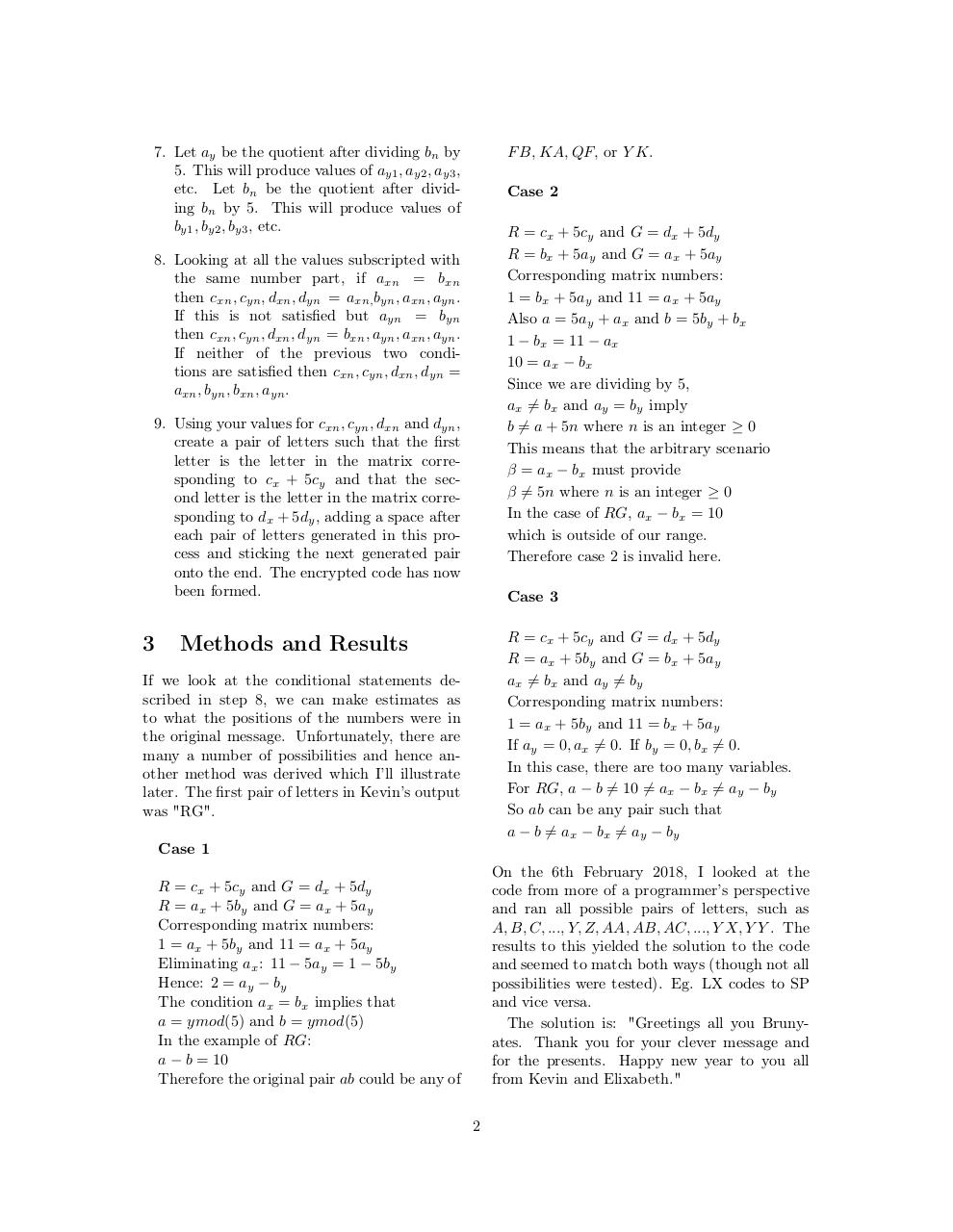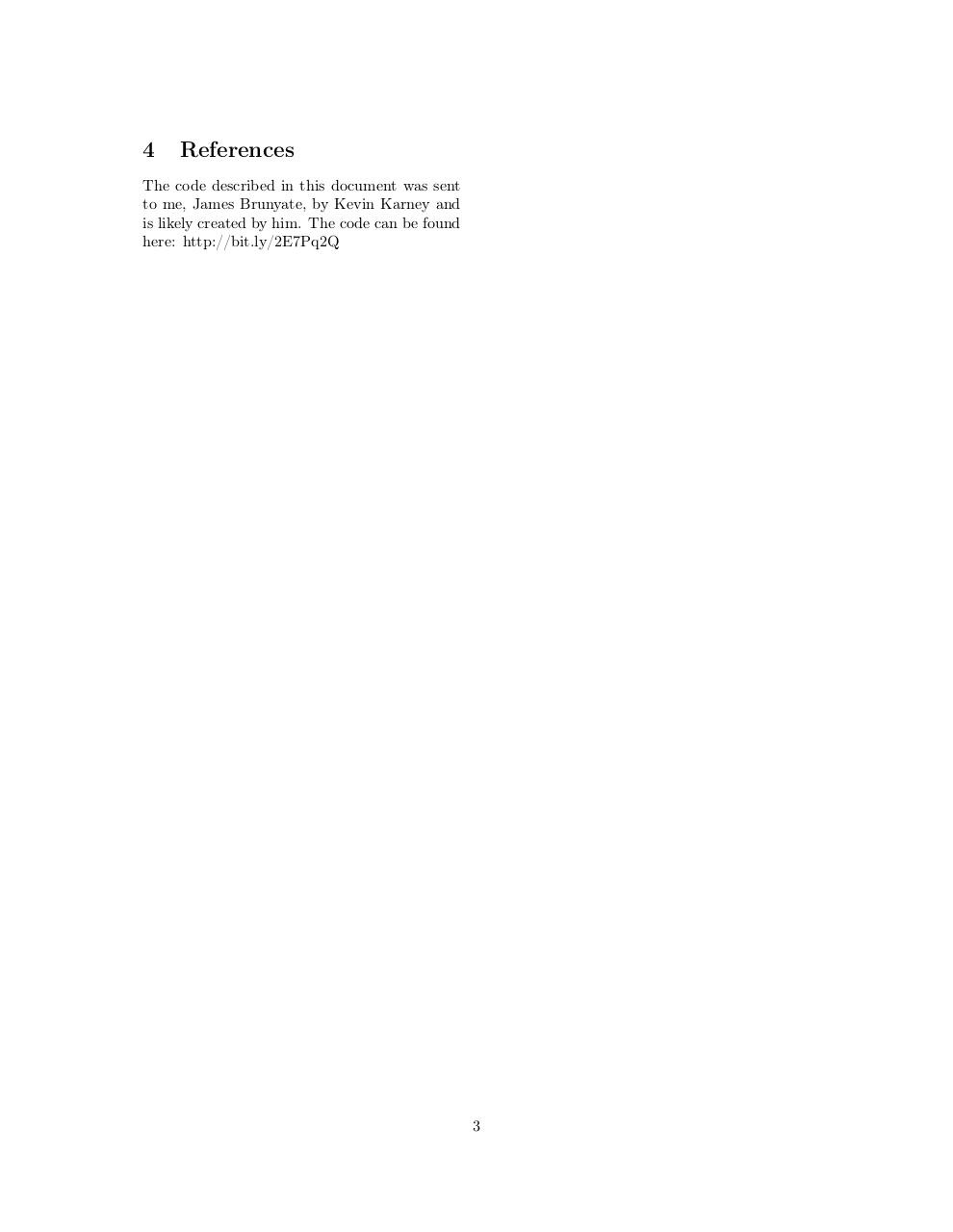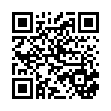# karney .pdf

### File information

Original filename: karney.pdf

This PDF 1.5 document has been generated by LaTeX with hyperref package / pdfTeX-1.40.17, and has been sent on pdf-archive.com on 07/02/2018 at 00:20, from IP address 137.222.x.x. The current document download page has been viewed 218 times.
File size: 135 KB (3 pages).
Privacy: public file

karney.pdf (PDF, 135 KB)

### Document preview

Decoding The Thank You Card
James Brunyate
Homies
jamespbrunyate@gmail.com

February 6, 2018

Abstract
1

YATECDFGHIJKLMOPQSVWX". This
list of letters shall be called the "matrix".

Introduction

to each letter in the matrix, convert every
letter in the original message to upper-case
and remove any spaces in the original message. If there is an odd number of letters
in the original message, add on an ’X’ to
the end of the message.

After receiving terrible gifts from the Karneys
for Christmas 2017, us squibs decided to send
the Karneys a thank you card explaining how
grateful we were for the gifts. We were, of
course, all lying. The thank you card we sent
was in the form of a cryptogram with two or
three letters given to start off with.
In late January, after some time had passed,
Kaija received an email from Kevin Karney
which basically was an encrypted message back
to us in the form of Python code... Annoyingly,
Kevin knows that only I am capable of discerning a lot of the Python that he was written and
so the task falls to me to decode it. It was become an occasional hobby for when I don’t feel
like doing anything else. I decided to document
this in a proper article form on Monday 5th
February 2018.
Kevin’s code uses a multitude of layers to encrypt any message he decides to send. His code
is not case-sensitive, does not contain punctuation and will not function properly if the character ’z’ is used. Hence his original message
does not contain the character ’z’ or any punctuation.

2

3. Count the number of letters now in the
message. Divide this number by two. This
will be our range.
4. For each number ’n’ from 0 to 24, find 2*n.
Numbering each letter in the message 0,
1, 2, 3, etc. Find the letter corresponding to 2n in the message. Using the numbers corresponding to the matrix, take the
letter we just obtained and find it’s corresponding number from that series of numbers. These numbers will be denoted by
a1 , a2 , a3 , etc.
5. Repeat the procedure for 2n+1 instead of
2n, denoting the final outcome values as
b1 , b2 , b3 , etc.
6. Let ax be the remainder after dividing
an by 5. This will produce values of
ax1 , ax2 , ax3 , etc. Let bx be the remainder
after dividing bn by 5. This will produce
values of bx1 , bx2 , bx3 , etc. Any value subscripted with an x will hence be between 0
and 4 since we are dividing by 5 and the
only possible remainders lie between 0 and
4 inclusive.

Code operations

1. Remove the following letters from the alphabet: B, R, U, N, Y, A, T, E and Z. Form
a list of letters in the order "B, R, U, N, Y,
A, T, E" and then add on all the remaining
letters of the alphabet to obtain "BRUN1

7. Let ay be the quotient after dividing bn by
5. This will produce values of ay1 , ay2 , ay3 ,
etc. Let bn be the quotient after dividing bn by 5. This will produce values of
by1 , by2 , by3 , etc.

F B, KA, QF, or Y K.
Case 2
R = cx + 5cy and G = dx + 5dy
R = bx + 5ay and G = ax + 5ay
Corresponding matrix numbers:
1 = bx + 5ay and 11 = ax + 5ay
Also a = 5ay + ax and b = 5by + bx
1 − bx = 11 − ax
10 = ax − bx
Since we are dividing by 5,
ax 6= bx and ay = by imply
b 6= a + 5n where n is an integer ≥ 0
This means that the arbitrary scenario
β = ax − bx must provide
β 6= 5n where n is an integer ≥ 0
In the case of RG, ax − bx = 10
which is outside of our range.
Therefore case 2 is invalid here.

8. Looking at all the values subscripted with
the same number part, if axn = bxn
then cxn , cyn , dxn , dyn = axn, byn , axn , ayn .
If this is not satisfied but ayn = byn
then cxn , cyn , dxn , dyn = bxn , ayn , axn , ayn .
If neither of the previous two conditions are satisfied then cxn , cyn , dxn , dyn =
axn , byn , bxn , ayn .
9. Using your values for cxn , cyn , dxn and dyn ,
create a pair of letters such that the first
letter is the letter in the matrix corresponding to cx + 5cy and that the second letter is the letter in the matrix corresponding to dx + 5dy , adding a space after
each pair of letters generated in this process and sticking the next generated pair
onto the end. The encrypted code has now
been formed.

3

Case 3
R = cx + 5cy and G = dx + 5dy
R = ax + 5by and G = bx + 5ay
ax 6= bx and ay 6= by
Corresponding matrix numbers:
1 = ax + 5by and 11 = bx + 5ay
If ay = 0, ax 6= 0. If by = 0, bx 6= 0.
In this case, there are too many variables.
For RG, a − b 6= 10 6= ax − bx 6= ay − by
So ab can be any pair such that
a − b 6= ax − bx 6= ay − by

Methods and Results

If we look at the conditional statements described in step 8, we can make estimates as
to what the positions of the numbers were in
the original message. Unfortunately, there are
many a number of possibilities and hence another method was derived which I’ll illustrate
later. The first pair of letters in Kevin’s output
was "RG".
Case 1

On the 6th February 2018, I looked at the
code from more of a programmer’s perspective
and ran all possible pairs of letters, such as
A, B, C, ..., Y, Z, AA, AB, AC, ..., Y X, Y Y . The
results to this yielded the solution to the code
and seemed to match both ways (though not all
possibilities were tested). Eg. LX codes to SP
and vice versa.
The solution is: "Greetings all you Brunyates. Thank you for your clever message and
for the presents. Happy new year to you all
from Kevin and Elixabeth."

R = cx + 5cy and G = dx + 5dy
R = ax + 5by and G = ax + 5ay
Corresponding matrix numbers:
1 = ax + 5by and 11 = ax + 5ay
Eliminating ax : 11 − 5ay = 1 − 5by
Hence: 2 = ay − by
The condition ax = bx implies that
a = ymod(5) and b = ymod(5)
In the example of RG:
a − b = 10
Therefore the original pair ab could be any of
2

4

References

The code described in this document was sent
to me, James Brunyate, by Kevin Karney and
is likely created by him. The code can be found
here: http://bit.ly/2E7Pq2Q

3#### HTML Code

Copy the following HTML code to share your document on a Website or Blog

#### QR Code### Related keywords